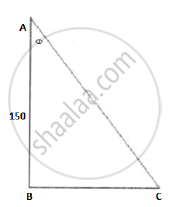# The angle of depression of a car parked on the road from the top of a 150 m high tower is 30°. The distance of the car from the tower (in metres) is - Mathematics

The angle of depression of a car parked on the road from the top of a 150 m high tower is 30°. The distance of the car from the tower (in metres) is

(A) 50sqrt3

(B) 150sqrt 3

(C) 150sqrt2

(D) 75

#### SolutionLet AB be the tower and BC be distance between tower and car. Let θ be the angle of depression of the car.
According to the given information,

In triangleABC,

tan theta =

BC=150/sqrt3=(150sqrt3)/3=50sqrt3

Hence, distance between the tower and car is  50 sqrt3

.

Concept: Heights and Distances
Is there an error in this question or solution?